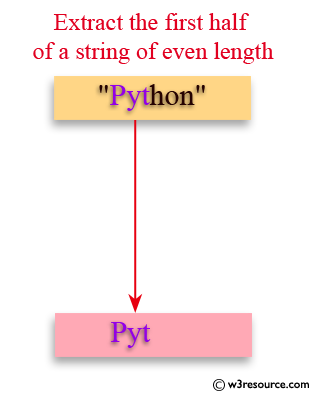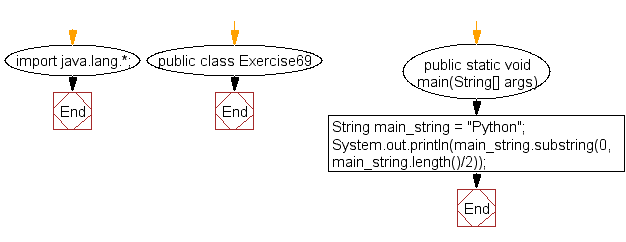﻿ Java exercises: Extract the first half of a string of even length - w3resource# Java Exercises: Extract the first half of a string of even length

## Java Basic: Exercise-69 with Solution

Write a Java program to extract the first half of a string of even length.

Pictorial Presentation:Sample Solution:

Java Code:

``````import java.lang.*;
public class Exercise69 {
public static void main(String[] args)
{
String main_string = "Python";
System.out.println(main_string.substring(0, main_string.length()/2));
}
}
```
```

Sample Output:

```Pyt
```

Flowchart:Java Code Editor:

What is the difficulty level of this exercise?

﻿

## Java: Tips of the Day

initial:

Returns all the elements of an array except the last one. Use Arrays.copyOfRange() to return all except the last one

```public static <T> T[] initial(T[] elements) {
return Arrays.copyOfRange(elements, 0, elements.length - 1);
}
```

Ref: https://bit.ly/3zEKw9u